# Shot Noise and Bit-Error Rate (BER) for Coherent Demodulation and Delay Demodulation

The signal-to-noise ratio (SNR) and the resulting BER for a specific modulation format depend on the demodulation scheme employed. This is so because the noise added to the signal is different for different demodulation schemes. In this tutorial we consider the shot-noise limit and discuss BER for the three demodulation schemes discussed in the demodulation schemes tutorial. In the next tutorial we will focus on a more realistic situation in which system performance is limited by other noise sources introduced by lasers and optical amplifiers employed along the fiber link.

Consider first the case of the binary ASK format. The signal used by the decision circuit is given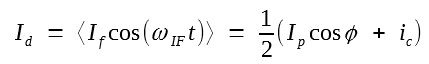with φ = 0. The phase difference φ = φs - φLO generally varies randomly because of phase fluctuations associated with the transmitter laser and the local oscillator. We consider such fluctuations later in the next tutorial but neglect them here as our objective is to discuss the shot-noise limit. The decision signal for the ASK format then becomes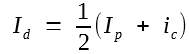where Ip ≡ 2Rd(PsPLO)1/2 takes values I1 and I0 depending on whether a 1 or 0 bit is being detected. We assume no power is transmitted during the 0 bits and set I0 = 0.

Except for the factor of 1/2 in this equation, the situation is analogous to the case of direct detection discussed in the optical receiver sensitivity tutorial. The factor of 1/2 does not affect the BER since both the signal and the noise are reduced by the same factor, leaving the SNR unchanged. In fact, one can use the same result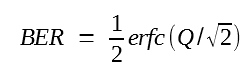where the Q factor can be written as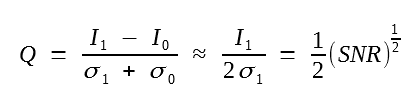In relating Q to SNR, we used I0 = 0 and set σ0 ≈ σ1. The latter approximation is justified for coherent receivers whose noise is dominated by shot noise induced by the local-oscillator and remains the same irrespective of the received signal power. As shown in the coherent detection tutorial, the SNR can be related to the number of photons Np received during each 1 bit by the simple relation SNR = 2ηNp, where η is the quantum efficiency of the photodetectors employed.

The use of the two equations above with SNR = 2ηNp provides the following expression for the BER:One can use the same method to calculate the BER in the case of ASK homodyne receivers. The last BER equation and the Q equation above still remain applicable. However, the SNR is improved by 3 dB in the homodyne case.

The last BER equation can be used to calculate the receiver sensitivity at a specific BER. Similar to the direct-detection case discussed in the optical receiver sensitivity tutorial, we define the receiver sensitivity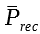as the average received power required for realizing a BER of 10-9 or less. From the two equations above, BER = 10-9 when Q ≈ 6, or when SNR = 144 (21.6 dB). We can use the following equation to relate SNR to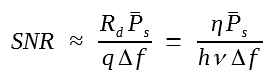if we note that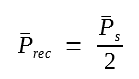simply because signal power is zero during the 0 bits. The result is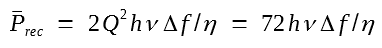In the ASK homodyne case,is smaller by a factor of 2 because of the 3-dB homodyne-detection advantage. As an example, for a 1.55-μm ASK heterodyne receiver with η = 0.8 and Δf = 1 GHz, the receiver sensitivity is about 12 nW and reduces to 6 nW if homodyne detection is used.

The receiver sensitivity is often quoted in terms of the number of photons Np using the BER expression above because such a choice makes it independent of the receiver bandwidth and the operating wavelength. Furthermore, η is also set to 1 so that the sensitivity corresponds to an ideal photodetector. It is easy to verify that for realizing a BER of 10-9, Np should be 72 and 36 in the heterodyne and homodyne cases, respectively. It is important to remember that Np corresponds to the number of photons within a single 1 bit. The average number of photons per bit,, is reduced by a factor of 2 in the case of binary ASK format.

Consider now the case of the BPSK format. The signal at the decision circuit is given by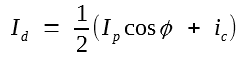The main difference from the ASK case is that Ip is  constant, but the phase φ takes values 0 or π depending on whether a 1 or 0 is being transmitted. In both cases, Id is a Gaussian random variable but its average value is either Ip/2 or - Ip/2, depending on the received bit. The situation is analogous to the ASK case with the difference that I0 = - I1 in place of being zero. In fact, we can usefor the BER, but Q is now given by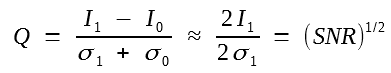where I0 = - I1 and σ0 = σ1 was used. By using SNR = 2ηNp, the BER is given byAs before, the SNR is improved by 3 dB, or by a factor of 2, in the case of PSK homodyne detection.

The receiver sensitivity at a BER of 10-9 can be obtained by using Q = 6. For the purpose of comparison, it is useful to express the receiver sensitivity in terms of the number of photons Np. It is easy to verify that Np = 18 and 9 for heterodyne and homodyne BPSK detection, respectively. The average number of photons/bit,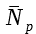, equals Np for the PSK format because the same power is transmitted during 1 and 0 bits. A PSK homodyne receiver is the most sensitive receiver, requiring only 9 photons/bit.

For completeness, consider the case of binary FSK format for which heterodyne receivers employ a dual-filter scheme, each filter passing only 1 or 0 bits. The scheme is equivalent to two complementary ASK heterodyne receivers operating in parallel. This feature allows us to usefor the FSK as well. However, the SNR is improved by a factor of 2 compared with the ASK case because the same amount of power is received even during 0 bits. By using SNR = 4ηNp, the BER is given by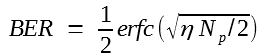In terms of the number of photons, the sensitivity is given byThe figure below shows the BER as a function of ηNp for the ASK, PSK, and FSK formats, demodulated by using a synchronous heterodyne receiver.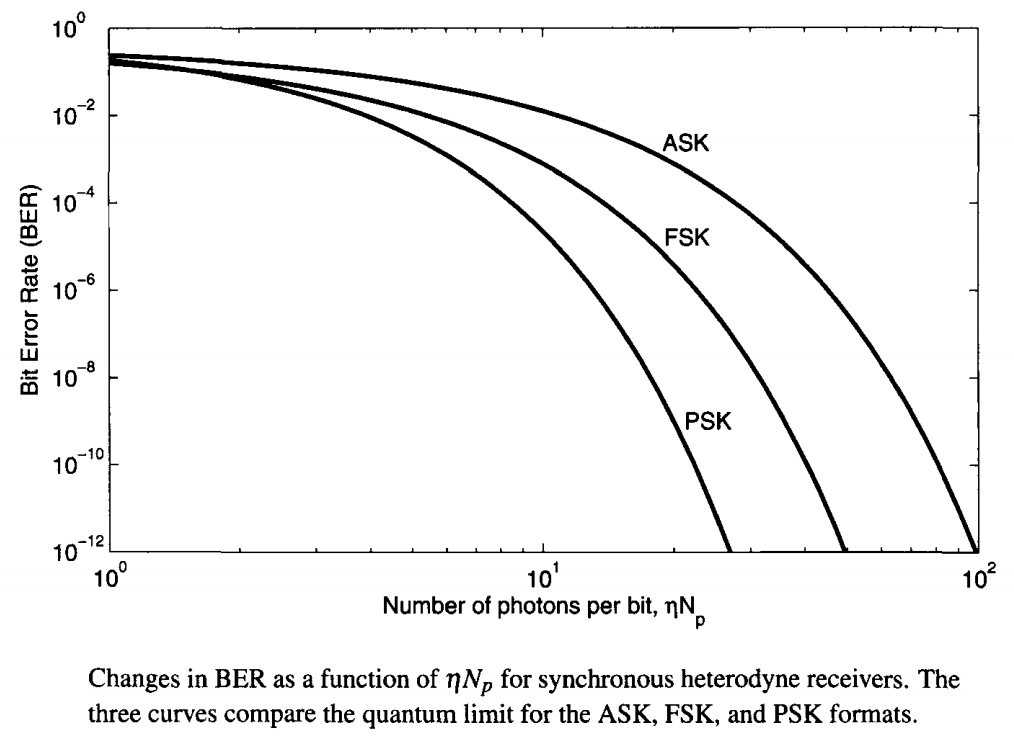It is interesting to compare the sensitivity of coherent receivers with that of a direct-detection receiver. The table below shows such a comparison.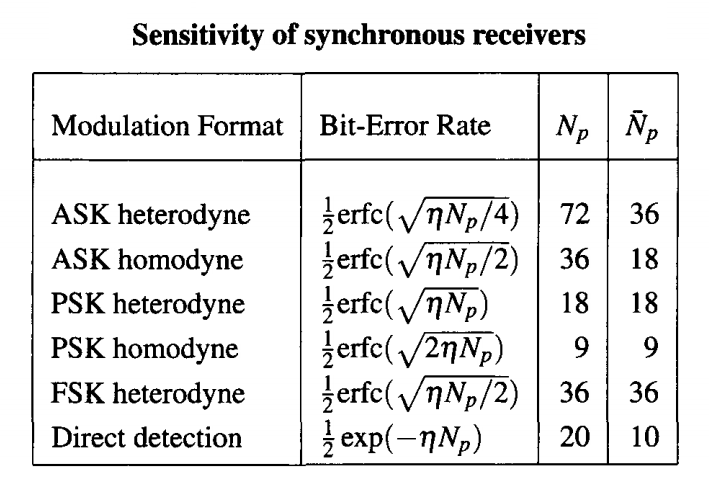As discussed in the optical receiver sensitivity tutorial, an ideal direct-detection receiver requires 10 photons/bit to operate at a BER of ≤ 10-9. This value is considerably superior to that of heterodyne schemes. However, it is never achieved in practice because of thermal noise, dark current, and many other factors, which degrade the sensitivity to the extent that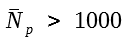is usually required. In the case of coherent receivers,below 100 can be realized because shot noise can be made dominant by increasing the local-oscillator power.

The BER calculation for asynchronous receivers is more complicated because the noise does not remain Gaussian when an envelop detector is used. The reason can be understood from this equation showing the signal processed by the decision circuit.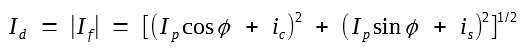In the case of an ideal ASK heterodyne receiver, φ can be set to zero so that (subscript d is dropped for simplicity)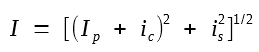Even though both ic and is are Gaussian random variable with zero mean and the same standard deviation σ, where σ is the root mean square (RMS) noise current, the probability density function (PDF) of I is not Gaussian. It can be calculated by using a standard technique and is found to be given by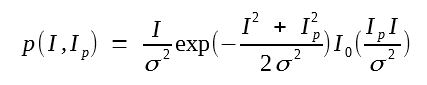where I0(x) represents a modified Bessel function of the first kind and I varies in the range 0 to ∞ because the output of an envelop detector can have only positive values. This PDF is known as the Rice distribution. When Ip = 0, the Rice distribution reduces to a Rayleigh distribution, well known in statistical optics.

The BER calculation follows the analysis of the optical receiver sensitivity tutorial with the only difference that the Rice distribution needs to be used in place of the Gaussian distribution. The BER is given bywith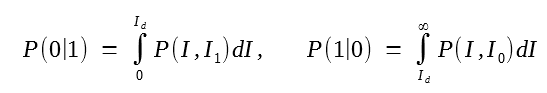where ID is the decision level and I1 and I0 are values of Ip for 1 and 0 bits. The noise is the same for all bits (σ0 = σ1 = σ) because it is dominated by the local oscillator power. The integrals in the equations can be expressed in terms of Marcum's Q function, defined as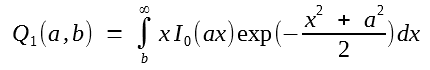The result for the BER isThe decision level ID is chosen such that the BER is minimum for given values of I1, I0, and σ. It is difficult to obtain an exact analytic expression of ID. However, under typical operating conditions, I0 ≈ 0, I1/σ ≫ 1, and ID is well approximated by I1/2. The BER then becomes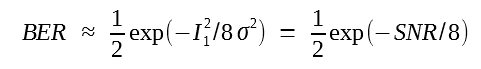Using SNR = 2ηNp, we obtain the final result,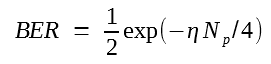A comparison with the following equation,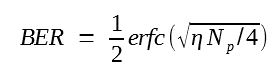obtained in the case of synchronous ASK receivers shows that the BER is larger in the asynchronous case for the same value of ηNp. However, the difference is so small that the receiver sensitivity at a BER of 10-9 is degraded by only 0.5 dB. If we assume η = 1,shows that BER = 10-9 forwhile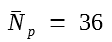in the synchronous case.

Consider next the PSK format. As mentioned earlier, asynchronous demodulation cannot be used for it. However, DBPSK signals can be demodulated by implementing the delay-demodulation scheme in the microwave regime. The filtered current in this following equation:is divided into two parts, and one part is delayed by exactly one symbol period Ts. The product of two currents depends on the phase difference between any two neighboring bits and is used by the decision current to determine the bit pattern.

To find the PDF of the decision variable, we write this equation in the form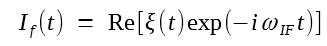where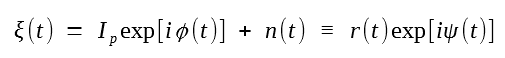Here, n = ic + iis is a complex Gaussian random process. The current used by the decision circuit can now be written asIf ωIFTs is chosen to be a multiple of 2π and we can approximate ψ with φ, then Id = ±r(t)r(t - Ts) as the phase difference takes its two values of 0 and π. The BER is thus determined by the PDF of the random variable r(t)r(t - Ts).

It is helpful to write this product in the form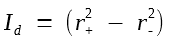where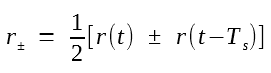Consider the error probability when φ = 0 for which Id > 0 in the absence of noise. An error will occur if r+ < r- because of noise. Thus, the conditional probability is given byThis probability can be calculated because the PDFs of r±2 can be obtained by noting that n(t) and n(t - Ts) are uncorrelated Gaussian random variables. The other conditional probability, P(0|π), can be found in the same manner. The final result is quite simple and is given byA BER of 10-9 is obtained for ηNp = 20. As a reminder, the quantity ηNp is just the SNR per bit in the shot noise limit.

#### 3. Receivers with Delay Demodulation

In the delay-demodulation scheme shown in the demodulation schemes tutorial, one or more MZ interferometers with one-symbol delay are used at the receiver end. In the DBPSK case, a single MZ interferometer is employed. The outputs of the two detectors in this case have average currents given byThe decision variable is formed by subtracting the two currents such that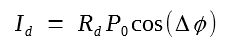The average currents for 0 and 1 bits are RdP0 and - RdP0 for Δφ = 0 and π, respectively.

To see how the noise affects the two currents, note that from this equation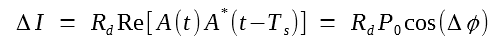that Id can be written in the form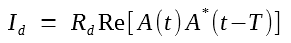whereis the optical field entering the receiver. Here, n(t) represent the noise induced by vacuum fluctuations that lead to the shot noise at the receiver. A comparison of this equation withobtained in the case of heterodyne detector with delay implemented in the microwave domain, shows the similarity between the two cases. Following the discussion presented here, one can conclude that the BER in the DBPSK case is again given byAs before, the SNR per bit, ηNp, sets the BER, and a BER of 10-9 is obtained for ηNp = 20.

The analysis is much more involved in the case of the DQPSK format. Proakis has developed a systematic approach for calculating error probabilities for a variety of modulation formats that includes the DQPSK format. Although his analysis is for a heterodyne receiver with delay implemented in the microwave domain, the results apply as well to the case of optical delay demodulation. In particular, when the DQPSK format is implemented with the Gray coding, the BER is given by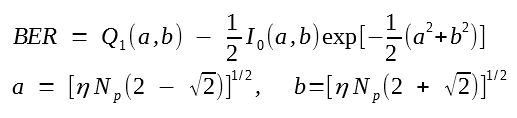where I0 is the modified Bessel function of order zero and Q1(a, b) is Marcum's Q function introduced earlier.

The following figure shows the BER curves for the DBPSK and DQPSK formats and compares them with the BER curve obtained in the case in which a heterodyne receiver is employed to detect the BPSK or the QPSK format (without differential encoding).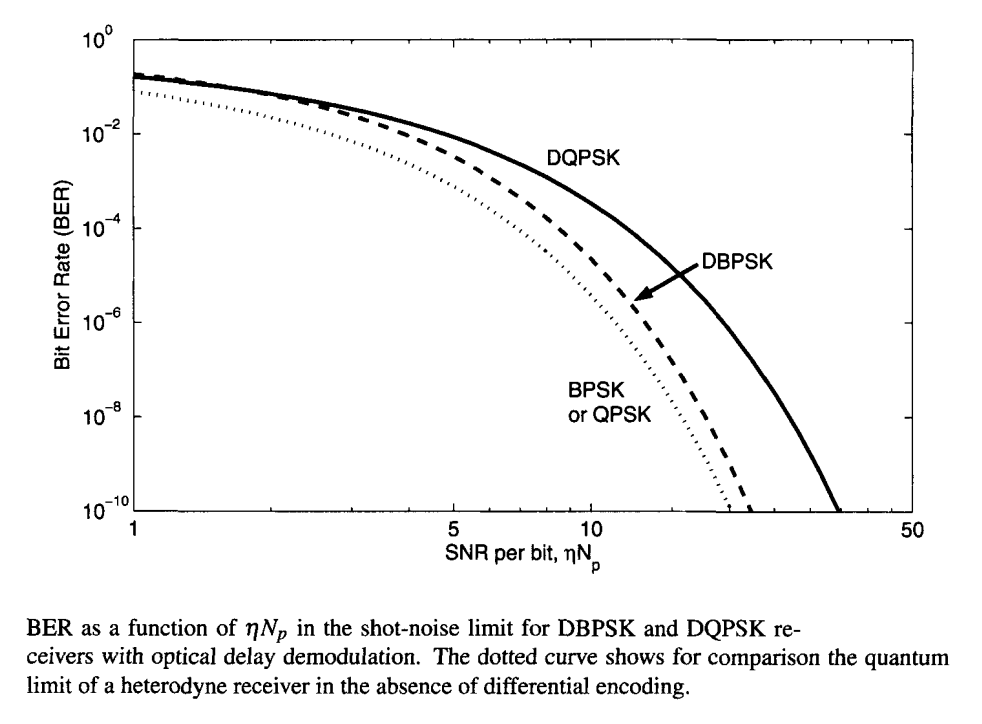When DBPSK is used in place of BPSK, the receiver sensitivity at a BER of 10-9 changes from 18 to 20 photons/bit, indicating a power penalty of less than 0.5 dB. In view of a such a small penalty, DBPSK is often used in place of BPSK because its use avoids the need of a local oscillator and simplifies the receiver design considerably. However, a penalty of close to 2.4 dB occurs in the case of DQPSK format for which receiver sensitivity changes from 18 to 31 photons/bit.

Because of the complexity of the BER expression above, it is useful to find its approximate analytic form. Using the upper and lower bounds on Marcum's Q function, this BER expression can be written in the following simple formThis expression is accurate to within 1% for BER values below 3 x 10-2. If we now employ asymptotic expansions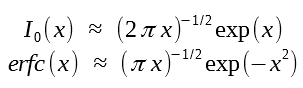valid for large values of x, and use a and b from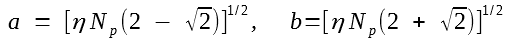we obtainThis expression is accurate to within a few percent for values of ηNp > 3.